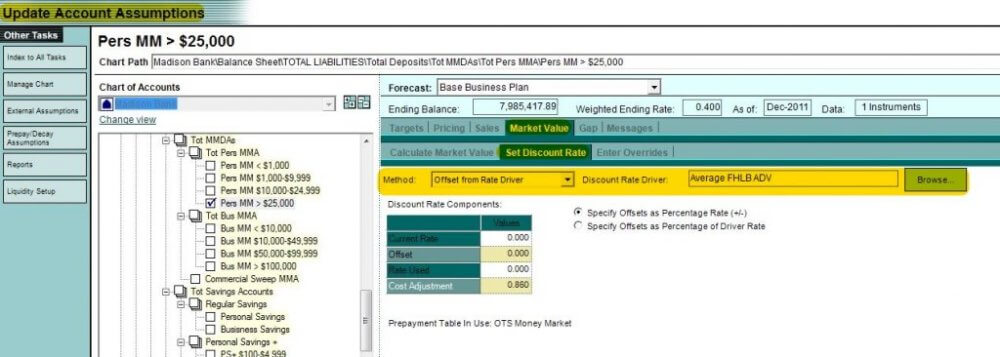Interest Rate Risk is the potential for loss of future income or economic value of equity caused by an adverse change in interest rates. If we go one step further, economic value analysis estimates the sensitivity or change in the economic value of equity to changes in interest rates. Depending on your charter this is Economic Value of Equity (EVE), Net Portfolio Value (NPV) or Net Economic Value (NEV) sensitivity. Economic value analysis uses the full cash flows of a financial institution’s assets and liabilities, so it summarizes information about expected earnings over a much longer time period than a 12 to 36 month income forecast. Thus, it is a measure of your long-term earnings capacity.

Because the future is uncertain, no one can consistently predict the future course of interest rates. The correct message from this analysis is that you should position your risk exposures so you can live with a wide range of interest rate changes. Modeling starts with net present value (NPV), which is the current value of all cash flows received in the future; calculated by discounting the cash flows at an appropriate interest rate, which is referred to as the discount rate.

The process of measuring IRR with economic value analysis is relatively straightforward. First determine the economic value of most assets and liabilities, at current market rates, which involves discounting the cash flows to estimate a net present value. The net present value of the assets and liabilities are then used to calculate the economic value of equity. Finally the same process is calculated again with increased or decreased market rates. Interest rate risk is measured by the change in the economic value of equity from the current rate to each of the increased or decreased market rates.

Running a current market value report is like the end of period balance sheet. The economic value analysis measures the risk from assets and liabilities currently on the balance sheet. Economic value of equity is a measure of a financial institution’s earning capacity and the analysis tries to determine how its earning capacity is affected by changes in interest rates. What this means is a current market value report is a static report and does not take into account any future new money or alternative forecast strategies.

With some of the basics understood let’s look at our responsibility in Foresight™ to model non-maturing deposits:

1. Cash Flows Since non-maturing deposits have no actual maturity dates, the model will need to calculate the duration or life of these accounts (i.e. an artificial maturity). This is accomplished by using a decay table provided by a Core Deposit Study, McGuire Performance Solutions decay tables, User Defined or potentially the old OTS tables.  To verify that the proper decay rate table is assigned to the non-maturing deposit go to Manage Chart of Accounts screen for an account and view the table selected on the Characteristics tab. The Prepayment/Decay Assumptions section of the model shows the detail on the decay tables by type of non-maturing deposit and rate changes. Viewing a GAP report will show you how the decay tables are spreading the NMD balances in the future.  These artificial maturities are then used in the present value calculation for Market Value.

2. Pricing Before market value is calculated the Pricing tab of the Update Account Assumptions screen must also be properly set. This setting is essential in the market value calculation because rate shocks not only adjust the market rate immediately, they also adjust the non-maturing deposit rate. For most institutions, there is a lag effect in changing NMD rates when market rates move. To verify this setting view the Lag Periods and the Apply% rates in Increases and Decreases on the Pricing screen.  The old OTS guidance on setting Reaction to Rate Shocks is as follows: Savings 33%, NOW 25% and MMDA 50% with each lag taking 6 months. A Core Deposit Study will provide both institution specific betas and lags at the product level. The results from the study will be incorporated into your model with the help of a Farin associate.

3. Discount Rate Driver Next we need to use a market curve to discount these future cash flows produced by the decay schedule. On the Market Value tab of the Update Account Assumptions screen you will find a Browse button next to the Discount Rate Driver selection. The driver we will use is the Average FHLB Advance curve since this is an alternative source of funding (i.e. market rate). There will be no additional Offset with this driver; therefore the Offset should be zero.

4. Cost Adjustment You also need to consider and adjust for the cost of servicing these non-maturing deposits. Besides the interest cost these deposits also have additional cost such as: mailing, supplies, personnel, hardware etc. While outdated, the old OTS average servicing cost for NMD’s are: NIB-Deposits 2.57%, Transaction Accounts (NOW) 1.80%, MMDA’s .86%, Passbook Accounts 1.39% and Certificates of Deposit’s .20%. These cost adjustments are entered on the Market Value tab for each non-maturing deposit. These cost adjustments are likely much lower and can be modified by the user./

*Some of the solutions or services mentioned in this post may no longer be offered. For information on alternatives, please go to visit Abrigo Advisory Services.​